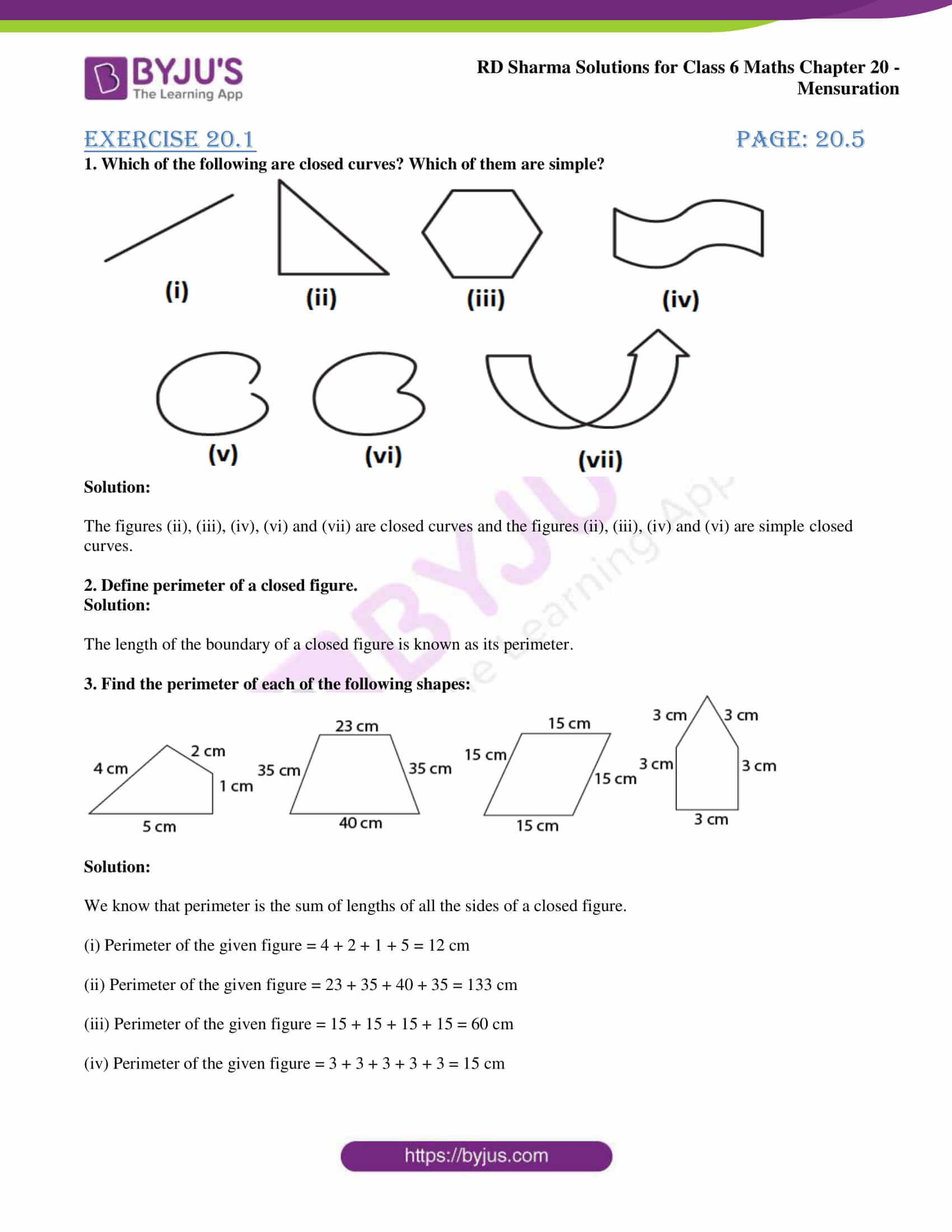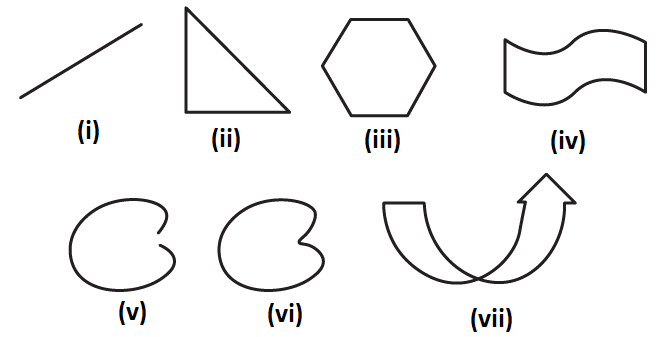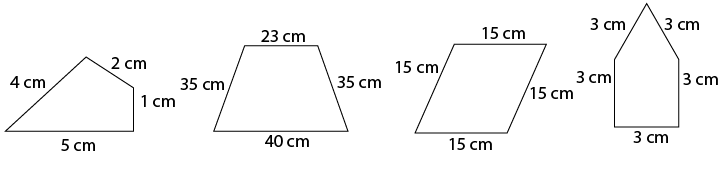# RD Sharma Solutions for Class 6 Maths Chapter 20: Mensuration Exercise 20.1

The first thing which comes to our mind when we think about plane figures are their regions and boundaries. Exercise 20.1 consists of problems which help students understand perimeter and length closed curves and regions. The solutions are relevant to RD Sharma textbook of the latest CBSE syllabus. The PDF can be downloaded by the students and used as a reference guide to improve their exam preparation. Students who aspire to obtain a good score in Mathematics can download RD Sharma Solutions Class 6 Maths Chapter 20 Mensuration Exercise 20.1 PDF which are available here.

## RD Sharma Solutions for Class 6 Maths Chapter 20: Mensuration Exercise 20.1 Download PDF### Exercise 20.1 page: 20.5

1. Which of the following are closed curves? Which of them are simple?Solution:

The figures (ii), (iii), (iv), (vi) and (vii) are closed curves and the figures (ii), (iii), (iv) and (vi) are simple closed curves.

2. Define perimeter of a closed figure.

Solution:

The length of the boundary of a closed figure is known as its perimeter.

3. Find the perimeter of each of the following shapes:Solution:

We know that perimeter is the sum of lengths of all the sides of a closed figure.

(i) Perimeter of the given figure = 4 + 2 + 1 + 5 = 12 cm

(ii) Perimeter of the given figure = 23 + 35 + 40 + 35 = 133 cm

(iii) Perimeter of the given figure = 15 + 15 + 15 + 15 = 60 cm

(iv) Perimeter of the given figure = 3 + 3 + 3 + 3 + 3 = 15 cm